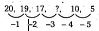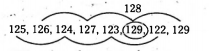# NMMS Scholarship Test November 2019 Previous Year Question Paper With Complete Analysis With Key Free Online Mock TestNMMS Scholarship Test November 2019 Previous Year Question Paper With Complete Analysis With Key Free Online Mock Test

National Means and Merit Scholarship Test
NOVEMBER 2019
Part -1 : Mental Ability Test

Directions :
Questions (1 to 5) : In the number series given below, one number is missing. Each series is followed by
4 alternatives (1) (2) (3), (4). One of them is the right answer. Identify and indicate it as per the “Instructions”.

1. 1, 4, 9, 16, 25, ?
1) 35
2) 36
3) 48
4) 49

Ans: 2) 36
Solution: 12 = 1, 22 = 4, 32 = 9, 42 = 16, 52 = 25
Similarly, 62 = 36

2. 20,19,17,?,10,5
1) 12
2) 13
3) 14
4) 15

Ans: 3) 14
Solution:So, 17-3= 14

3. 2, 3, 5, 7, 11.?, 17
1) 12
2) 13
3) 14
4) 15

Ans: 2) 13
Solution: Above all numbers are prime numbers 2,3, 5, 7, 11, 13, 17.

4. 165, 195, 255, 285, 345, ?
1) 375
2) 420
3) 435
4) 390

Ans: 1) 375
Solution: 165 + 30 = 195,
195 + 60 = 255,
255 + 30 = 285,
285 + 60 = 345,
345 + 30 = 375

5. 5, 17, 37, 65, ?,145
1) 95
2) 97
3) 99
4) 101

Ans: 4) 101
Solution: The series follows the pattern of
22 + 1, 42 + 1, 62 + 1, 82 + 1, …….
Similarly, 102 + 1 = 100 + 1 = 101

Directions : ‘
Questions (6 to 10) : In the following questions, one term in number series is incorrect. Find out the incorrect number.

6. 105,85,60,30,0,-45,-90
1) 105
2) 60
3) 0
4) -45

Ans: 3) 0
Solution: Here the sequence follows the terms – 20, – 25, – 30…….
So, 0 is wrong and must be replaced by (30 – 35). i.e., – 5

7. 325, 259, 202, 160, 127, 105, 94
1) 94
2) 127
3) 202
4) 259

Ans: 3) 202
Solution: Here the sequence follows the terms
-66,-55,-44,-33,-22,- 11
i.e.,
259 – 325 = – 66
.
.
.
127 – 160 = -33
105 -127 = -22
94- 105 = – 11
So, 202 is wrong and must be replaced by (259 -55). i.e., .204

8. 125, 126, 124, 127, 123, 129
1) 126
2) 124
3) 123
4) 129

Ans: 4) 129
Solution:9. 2, 5, 10, 50, 500, 5000
1) 5
2) 10
3) 50
4) 5000

Ans: 4) 5000
Solution: 2 x 5 = 10,
5 x 10 = 50,
10 x 50 = 500,
50 x 500 = 25000
Here the series follows, the product of preceding two terms. Here, the product of 50 x 500 = 25000.
So 5000 is wrong.

10. 46080, 3840, 384, 48, 24, 2, 1
1) 384
2) 48
3) 24
4) 2